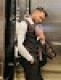# Trailing Zeros in 100 Factorial

Question: How many zeros are there in 100! (100 factorial)?

Answer: For those who don’t know what factorial is, 100! = 100 * 99 * 98 * … * 2 * 1

Ok, let’s look at how trailing zeros are formed in the first place. A trailing zero is formed when a multiple of 5 is multiplied with a multiple of 2. Now all we have to do is count the number of 5’s and 2’s in the multiplication.

Let’s count the 5’s first. 5, 10, 15, 20, 25 and so on making a total of 20. However there is more to this. Since 25, 50, 75 and 100 have two 5’s in each of them (25 = 5 * 5, 50 = 2 * 5 * 5, …), you have to count them twice. This makes the grand total 24. For people who like to look at it from a formula point of view

Number of 5’s = 100/5 + 100/25 + 100/125 + … = 24 (Integer values only)

Moving on to count the number of 2’s. 2, 4, 6, 8, 10 and so on. Total of 50 multiples of 2’s, 25 multiples of 4’s (count these once more), 12 multiples of 8’s (count these once more) and so on… The grand total comes out to

Number of 2’s = 100/2 + 100/4 + 100/8 + 100/16 + 100/32 + 100/64 + 100/128 + … = 97 (Integer values only)

Each pair of 2 and 5 will cause a trailing zero. Since we have only 24 5’s, we can only make 24 pairs of 2’s and 5’s thus the number of trailing zeros in 100 factorial is 24.

If you have any questions, please feel free to send me an email at [email protected]. If you have any interview questions which you feel would benefit others, I would love to hear about it.

If you like this post, please Digg it or give it a thumbs up on StumbleUpon.

If you're looking for some serious preparation for your interviews, I'd recommend this book written by a lead Google interviewer. It has 189 programming questions and solutions:## 6 Responses

1.Noor says:

How to count the number of trailing zero in a factorial in 5 seconds, watch

2.Sambhav Jain says:

What about 10, 20, 30 and so on which are also contributing zeroes in 100!

3.Noor says:

If N is a number then number of trailing zeroes in N! is

`N/5 + N/5^2 + N/5^3 ….. N/5^(m-1) WHERE (N/5^m)<1`

You can learn how this formula comes here at https://www.youtube.com/watch?v=wdz_KouqHx4

4.pratik_nalage says:

Going with your formula, if the input number is greater than 0.1 million then one can get wrong result.

XHTML: These are some of the tags you can use: `<a href=""> <b> <blockquote> <code> <em> <i> <strike> <strong>`